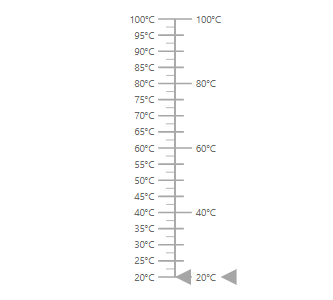Search results

# Axes in Blazor Linear Gauge component

28 Jul 2021 / 5 minutes to read

Axes is a collection of linear axis which can be used to indicate the numeric values. Line, ticks, labels, ranges and pointers are the sub elements of an axis.

## Line Customization

The `LinearGaugeLine` tag of an axis provides options to customize the `Height``Width`,`Color` and `Offset` of the axis line.

``````<SfLinearGauge>
<LinearGaugeAxes>
<LinearGaugeAxis>
<LinearGaugeLine Height="150"
Width="2"
Color="#4286f4"
Offset="2">
</LinearGaugeLine>
</LinearGaugeAxis>
</LinearGaugeAxes>
</SfLinearGauge>``````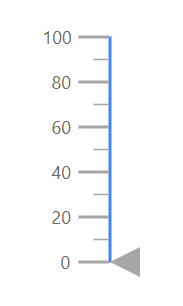## Ticks Customization

You can customize the `Height`, `Color` and `Width` of major and minor ticks, by using `LinearGaugeMajorTicks` and `LinearGaugeMinorTicks` tag. By default, interval for major ticks will be calculated automatically and also you can customize the interval for major and minor ticks using `Interval` property.

``````<SfLinearGauge>
<LinearGaugeAxes>
<LinearGaugeAxis Minimum =20 Maximum =140>
<LinearGaugeMajorTicks Color="#9e9e9e" Interval="20" Height="20">
</LinearGaugeMajorTicks>
<LinearGaugeMinorTicks Color="red" Interval="5" Height="10">
</LinearGaugeMinorTicks>
</LinearGaugeAxis>
</LinearGaugeAxes>
</SfLinearGauge>``````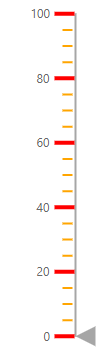Tick Position

The minor and major ticks can be positioned by using `Offset` and `Position` property. The offset defines the difference between the axis and the ticks. By default, the offset value is 0. The `Position` specifies the position of the ticks. Its possible values are ‘Inside’, ‘Outside’ and ‘Cross’. By default, ticks will be placed inside the axis.

``````<SfLinearGauge>
<LinearGaugeAxes>
<LinearGaugeAxis Minimum="0" Maximum="160">
<LinearGaugeMajorTicks Interval="10" Color="red" Height="10" Width="3" Position="Position.Outside">
</LinearGaugeMajorTicks>
<LinearGaugeMinorTicks Interval="5" Color="green" Height="5" Width="2" Position="Position.Cross">
</LinearGaugeMinorTicks>
</LinearGaugeAxis>
</LinearGaugeAxes>
</SfLinearGauge>``````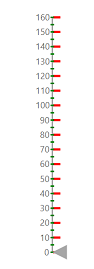## Labels Customization

Labels can be customized using the folllowing properties in `LinearGaugeAxisLabelStyle`

``````<SfLinearGauge>
<LinearGaugeAxes>
<LinearGaugeAxis>
<LinearGaugeAxisLabelStyle>
<LinearGaugeAxisLabelFont Color="red"></LinearGaugeAxisLabelFont>
</LinearGaugeAxisLabelStyle>
</LinearGaugeAxis>
</LinearGaugeAxes>
</SfLinearGauge>``````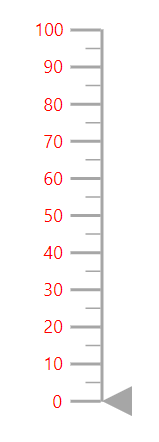Label Position

Labels can be positioned by using `Offset` or `Position` property. The `Offset` defines the distance between the labels and ticks. By default, the offset value is 0. The `Position` specifies the label position. Its possible values are ‘Inside’, ‘Outside’ and ‘Cross’. By default, labels will be placed `Inside` the axis.

``````<SfLinearGauge>
<LinearGaugeAxes>
<LinearGaugeAxis>
<LinearGaugeAxisLabelStyle Position="Position.Cross">
</LinearGaugeAxisLabelStyle>
</LinearGaugeAxis>
</LinearGaugeAxes>
</SfLinearGauge>``````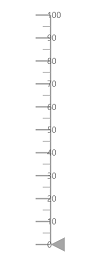Customize the display of the last label

If the last label is not in the visible range, it will be hidden by default. If you want to show the last label, set the `ShowLastLabel` property to true in the `LinearGaugeAxis`.

``````<SfLinearGauge>
<LinearGaugeAxes>
<LinearGaugeAxis Minimum="0" Maximum="170" ShowLastLabel="true">
</LinearGaugeAxis>
</LinearGaugeAxes>
</SfLinearGauge>``````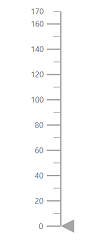Label Format

Axis labels in the Linear Gauge control can be formatted using the `Format` property in the `LinearGaugeAxisLabelStyle` class. It is used to render the axis labels in a certain format or to add a user-defined unit in the label. It works with the help of placeholder like {value}°C, where “value” represents the axis value. e.g. 20°C.

``````<SfLinearGauge>
<LinearGaugeAxes>
<LinearGaugeAxis Minimum =20 Maximum =140>
<LinearGaugeLabel Format= "{value}°C">
</LinearGaugeLabel>
</LinearGaugeAxis>
</LinearGaugeAxes>
</SfLinearGauge>``````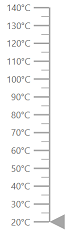Displaying numeric format in labels

The numeric formats such as currency, percentage, and so on can be displayed in the labels of the Linear Gauge using the `Format` property in the `SfLinearGauge` class. The following table describes the result of applying some commonly used label formats on numeric values.

 Label Value Label Format property value Result Description 1000 n1 1000.0 The number is rounded to 1 decimal place 1000 n2 1000.00 The number is rounded to 2 decimal place 1000 n3 1000.000 The number is rounded to 3 decimal place 0.01 p1 1.0% The number is converted to percentage with 1 decimal place 0.01 p2 1.00% The number is converted to percentage with 2 decimal place 0.01 p3 1.000% The number is converted to percentage with 3 decimal place 1000 c1 0. ,000.0 The currency symbol is appended to number and number is rounded to 1 decimal place 1000 c2 0. ,000.00 The currency symbol is appended to number and number is rounded to 2 decimal place
``````@using Syncfusion.Blazor.LinearGauge

<SfLinearGauge Format="c">
<LinearGaugeAxes>
<LinearGaugeAxis>
</LinearGaugeAxis>
</LinearGaugeAxes>
</SfLinearGauge>``````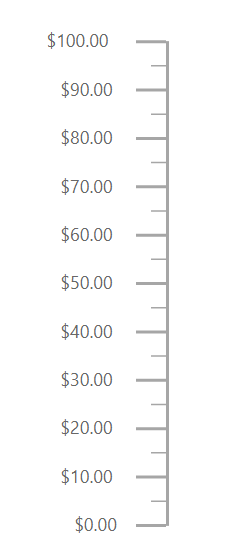## Orientation

By default, the Linear Gauge is rendered vertically. To change its orientation, the `Orientation`property must be set “Horizontal”

``````<SfLinearGauge Orientation="Orientation.Horizontal">
<LinearGaugeAxes>
<LinearGaugeAxis Minimum="20" Maximum="140"></LinearGauge>
</LinearGaugeAxes>
</SfLinearGauge>``````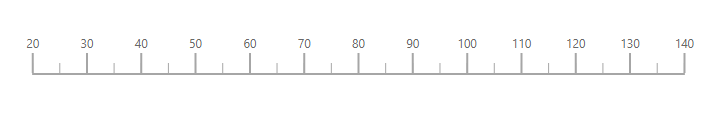## Inverted Axes

`IsInversed` property is used to choose the rendering of axis either bottom to top or top to bottom direction.

``````<SfLinearGauge>
<LinearGaugeAxes>
<LinearGaugeAxis IsInversed=true>
</LinearGaugeAxis>
</LinearGaugeAxes>
</SfLinearGauge>``````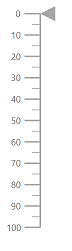## Opposed Axes

To place an axis opposite from its original position, set `OpposedPosition` property as true in the axis.

``````<SfLinearGauge>
<LinearGaugeAxes>
<LinearGaugeAxis OpposedPosition=true>
</LinearGaugeAxis>
</LinearGaugeAxes>
</SfLinearGauge>``````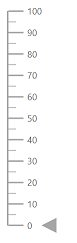## Multiple Axes

You can render any number of axis for a Linear Gauge by using array of axis objects. Each axis will have its own ranges, pointers, annotations and customization options.

``````<SfLinearGauge>
<LinearGaugeAxes>
<LinearGaugeAxis Minimum=20 Maximum=100>
<LinearGaugeLabel Format="{value}°C">
</LinearGaugeLabel>
</LinearGaugeAxis>
<LinearGaugeAxis Minimum=20 Maximum=100 OpposedPosition=true>
<LinearGaugeMajorTicks Interval="20" Height="20">
</LinearGaugeMajorTicks>
<LinearGaugeMinorTicks Interval="5" Height="10">
</LinearGaugeMinorTicks>
<LinearGaugeLabel Format="{value}°C">
</LinearGaugeLabel>
</LinearGaugeAxis>
</LinearGaugeAxes>
</SfLinearGauge>``````